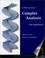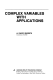### Complex Analysis

#### Course Description

Functions of complex variables. Analytic functions. Multiple-valued functions. Elementary functions. Conformal mappings. Möbius transform. Integral of functions of complex variable. Taylor and Laurent series. Zeroes and singular points of analytic functions. Residue theorem and applications. Inverse of Laplace transform. Special functions. Gamma and beta functions.

#### General Competencies

Learning advanced complex calculus and it’s use in various applications: conformal mappings, integral transforms, orthogonal polynomials and special functions.

#### Learning Outcomes

1. operate with elementary functions in complex domain
2. define and examine analytic property of functions
3. apply the technique of conformal mappings in analyze of simple models
4. use the technique of power series expansion in the calculus with elementary functions
5. classify zeroes and singular points of analytic functions
6. apply the technique of residuum, especially in the inverse integral transformation
7. use the properties of gamma and beta function in various situations

#### Forms of Teaching

Lectures

Lectures are organized through two cycles. The first cycle consists of 7 weeks of classes and mid-term exam, a second cycle of 6 weeks of classes and final exam. Classes are conducted through a total of 15 weeks with a weekly load of 3 hours.

Exams

Mid-term exam in the 8th week of classes and final exam in the 15th week of classes.

Consultations

Consultations are held one hour weekly according to arrangement with students.

Continuous Assessment Exam
Type Threshold Percent of Grade Threshold Percent of Grade
Mid Term Exam: Written 0 % 50 % 0 %
Final Exam: Written 0 % 50 %
Exam: Written 0 % 100 %

#### Week by Week Schedule

1. Complex plane. Regions. Contours.
2. Functions of complex variables. Multiple-valued functions. The power and root functions.
3. Exponential and logarithmic function. Trigonometric and other elementary functions.
4. Differentiability. Analytic functions. Cauchy-Riemann conditions. Harmonic functions.
5. Bilinear transformation (Möbius transform) and examples.
6. Conformal mappings. Examples of region mappings.
7. Integral of functions of complex variable. Independence of path. Cauchy theorem. Cauchy integral formula. Applications.
8. Exam
9. Taylor series. Zeroes of analytic functions.
10. Laurent series. Singular points and poles of analytic functions.
11. Residue calculus and residue theorem.
12. Applications of residue. Inverse Laplace transform.
13. Special functions. Gamma function.
14. Beta function. Applications.
15. Exam.

#### Study Programmes

[FER2-HR] Computer Engineering - profile
Mathematics and Science (1. semester)
[FER2-HR] Computer Science - profile
Mathematics and Science (1. semester)
[FER2-HR] Control Engineering and Automation - profile
Mathematics and Science (1. semester)
[FER2-HR] Electrical Engineering Systems and Technologies - profile
Mathematics and Science (1. semester)
[FER2-HR] Electrical Power Engineering - profile
Mathematics and Science (1. semester)
[FER2-HR] Electronic and Computer Engineering - profile
Mathematics and Science (1. semester)
[FER2-HR] Electronics - profile
Mathematics and Science (1. semester)
[FER2-HR] Information Processing - profile
Mathematics and Science (1. semester)
[FER2-HR] Software Engineering and Information Systems - profile
Mathematics and Science (1. semester)
[FER2-HR] Telecommunication and Informatics - profile
Mathematics and Science (1. semester)
[FER2-HR] Wireless Technologies - profile
Mathematics and Science (1. semester)

#### Literature

N. Elezović (2010.), Funkcije kompleksne varijable, ElementD. G. Zill, P. D. Shanahan (2003.), A First Course in Complex Analysis with Applications, Jones and BartlettA. D. Wunsch (1994.), Complex variables with Applications, Addison-Wesley

#### General

ID 34552
Winter semester
4 ECTS
L1 English Level
L1 e-Learning
45 Lectures
0 Seminar
0 Exercises
0 Laboratory exercises
0 Project laboratory